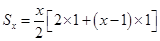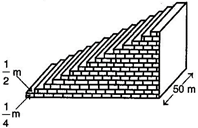NCERT Solutions Maths Class 10 Chapter 5

# NCERT Solutions Maths Class 10 Chapter 5

## NCERT Solutions Maths Class 10 Exercise 5.4

1. Which term of the AP: 121, 117, 113, …. is its first negative term?

Solution: The given AP is 121, 117, 113, …

Here, first term (a) = 121, common difference (d) = 117 – 121 = –4

Using formula, an = a + (n – 1)d, to find the nth term of an AP, we have

an = 121 + (n – 1)( –4)

= 121 – 4n + 4

= 125 – 4n

For the first negative term, an ˂ 0

125 – 4n ˂ 0

125 ˂ 4n

4n > 125

n > 125/4

n > 31.25

Since n is an integer and it is greater than 31.25, n = 32.

Hence, the first negative term is 32nd term.

2. The sum of the third and the seventh terms of an AP is 6 and their product is 8. Find the sum of first sixteen terms of the AP.

Solution: Let the given AP be a – 4d, a – 3d, a – 2d, ad, a, a + d, a + 2d, a + 3d, …

Then, a3 = a – 2d, a7 = a + 2d

According to the first condition, we have

a3 + a7 = 6

a – 2d + a + 2d = 6

2a = 6

a = 3 … (1)

According to the second condition, we have

(a – 2d) (a + 2d) = 8

a2 – 4d2 = 8

4d2 = a2 – 8

4d2 = 32 – 8

4d2 = 1

d2 = ¼

d = ± ½

Taking d = 1/2,

= 8(2 + 15/2)

= 8 × 19/2

= 76

Taking d = –1/2,= 8 × 5/2

= 20

Therefore, S16 = 20 or 76.

3. A ladder has rungs 25 cm apart (see the given figure). The rungs decrease uniformly in length from 45 cm at the bottom to 25 cm at the top. If the top and the bottom rungs are 2½ m apart, what is the length of the wood required for the rungs?

Solution: Number of rungs (n) = (2½ × 100)/25 = 10

The length of the wood required for rungs = sum of the 10 rungs

= (n/2)[a + l]

= (10/2)[25 + 45]

= 5 × 70

= 350 cm

4. The houses of a row are numbered consecutively from 1 to 49. Show that there is a value of x such that the sum of the numbers of the houses preceding the house numbered x is equal to the sum of the numbers of the houses following it. Find this value of x.

Solution: Here a =1 and d =1= (49/2)[2 + 48]

= 49 × 25

According to the question, we have

Sx – 1 = S49 Sx

x2 = 49 × 25

x = ±35

Since x is a counting number, therefore, we discard the negative value of x.

Hence, x = 35

5. A small terrace at a football ground comprises of 15 steps each of which is 50 m long and built of solid concrete.

Each step has a rise of 1/4 m and a tread of 1/2 m (see the given figure). Calculate the total volume of concrete required to build the terrace.Solution: The volume of concrete required to build the first step, second step, third step, … (in m2) are= (50/8) × (15/2) × 16

= 750 m3

Hence, the total volume of concrete required to build the terrace is 750 m3.